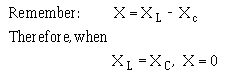apparent power is the power that must be provided to the circuit. You also know that the true power is the power actually used in the circuit. The difference between apparent power and true power is wasted because, in reality, only true power is consumed. The ideal situation would be for apparent power and true power to be equal.">Custom SearchPower Factor Correction The apparent power in an ac circuit has been described as the power the source "sees". As far as the source is concerned the apparent power is the power that must be provided to the circuit. You also know that the true power is the power actually used in the circuit. The difference between apparent power and true power is wasted because, in reality, only true power is consumed. The ideal situation would be for apparent power and true power to be equal. If this were the case the power factor would be 1 (unity) or 100 percent. There are two ways in which this condition can exist. (1) If the circuit is purely resistive or (2) if the circuit "appears" purely resistive to the source. To make the circuit appear purely resistive there must be no reactance. To have no reactance in the circuit, the inductive reactance (XL) and capacitive reactance (XC) must be equal.The expression "correcting the power factor" refers to reducing the reactance in a circuit. The ideal situation is to have no reactance in the circuit. This is accomplished by adding capacitive reactance to a circuit which is inductive and inductive reactance to a circuit which is capacitive. For example, the circuit shown in figure 4-10 has a total reactance of 80 ohms capacitive and the power factor was .6 or 60 percent. If 80 ohms of inductive reactance were added to this circuit (by adding another inductor) the circuit would have a total reactance of zero ohms and a power factor of 1 or 100 percent. The apparent and true power of this circuit would then be equal. Q.30 An ac circuit has a total reactance of 10 ohms inductive and a total resistance of 20 ohms. The power factor is .89. What would be necessary to correct the power factor to unity?Integrated Publishing, Inc. - A (SDVOSB) Service Disabled Veteran Owned Small Business